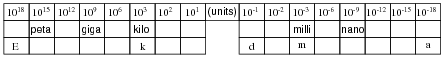# Scientific Notation and Metric Prefixes

## Mathematics for Electronics

• #### Question 1

Write the following exponential expressions in expanded form:

 101 =

 102 =

 103 =

 104 =

 10−1 =

 10−2 =

 10−3 =

 10−4 =

• #### Question 2

Evaluate the following arithmetic expressions, expressing the answers in expanded form:

 3.6 ×102 =

 1.53 ×10−4 =

 8.2 ×101 =

 6 ×10−3 =

Comment on the effect multiplication by a power of ten has on how a number is written.

• #### Question 3

Express the following numbers in scientific notation:

 0.00045 =

 23,000,000 =

 700,000,000,000 =

 0.00000000000000000001 =

 0.000098 =

• #### Question 4

Metric prefixes are nothing more than “shorthand” representations for certain powers of ten. Express the following quantities of mass (in units of grams) using metric prefixes rather than scientific notation, and complete the “index” of metric prefixes shown below:

 8.3 ×1018 g =

 3.91 ×1015 g =

 5.2 ×1012 g =

 9.3 ×109 g =

 6.7 ×106 g =

 6.8 ×103 g =

 4.5 ×102 g =

 8.11 ×101 g =

 2.1 ×10−1 g =

 1.9 ×10−2 g =

 9.32 ×10−3 g =

 1.58 ×10−6 g =

 8.80 ×10−9 g =

 6.9 ×10−12 g =

 7.2 ×10−15 g =

 4.1 ×10−18 g =• #### Question 5

What is the difference between the following three values of mass (in kilograms)?

 4 ×102 kg

 4.5 ×102 kg

 4.500 ×102 kg